3.MD.D.8 Test 7C Solve problems involving perimeters of polygons
 Name:    3.MD.D.8 Test 7C Solve problems involving perimeters of polygons

Multiple Choice
Identify the choice that best completes the statement or answers the question.

1.

What is the perimeter of this polygon?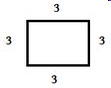a. 15 c. 3 b. 12 d. 9

2.

Both of these polygons have the same areas.  Which one has the larger perimeter?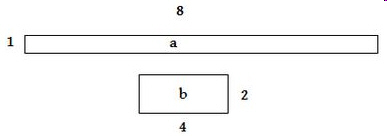a. a c. They are the same b. b

3.

The doghouse Peter built looked like this.  What is the perimeter of  the front wall of the dog house?a. 21 c. 15 b. 10.5 d. 17

4.

Peter was making a cement pad on which to build a dog house.  He used 8 foot  2” x 4” boards to frame out (put border around) the pad.     What is the perimeter of the pad for the dog house?a. 32 c. 24 b. 30 d. 16

5.

Alex decided to build his kids a sandbox in the yard.  What is the perimeter of the sandbox?a. 18 c. 36 b. 27 d. 9

6.

What is the perimeter of the white polygon?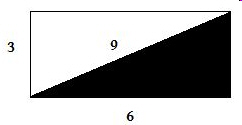a. 18 c. 20 b. 15 d. 12

7.

What is the perimeter of this polygon?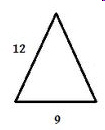a. 21 c. 3 b. 33 d. 24

8.

What is the perimeter of this polygon?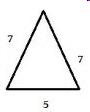a. 12 c. 14 b. 16 d. 19

9.

What is the perimeter of this polygon?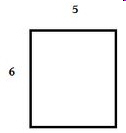a. 15 c. 11 b. 22 d. 20

10.

What is the perimeter of this polygon?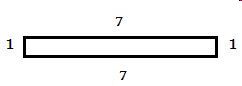a. 51 c. 14 b. 19 d. 16

11.

What is the perimeter of this polygon?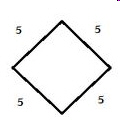a. 15 c. 20 b. 10 d. 5

12.

Both of these polygons have the same perimeter.  Which one has the larger area?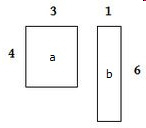a. a c. They are the same b. b